Next: Wave Mechanics Up: Classical Mechanics Previous: Hamilton's Principle

# Hamilton's Equations

Consider a dynamical system with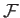degrees of freedom that is described by the generalized coordinates, for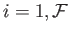. Suppose that neither the kinetic energy,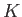, nor the potential energy,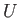, depend explicitly on the time,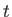. In conventional dynamical systems, the potential energy is generally independent of the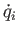, whereas the kinetic energy takes the form of a homogeneous quadratic function of the. In other words,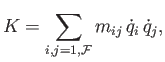(B.68)

where the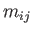depend on the, but not on the. It is easily demonstrated from the previous equation that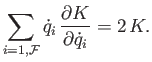(B.69)

Recall, from Section B.4, that generalized momentum conjugate to the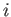th generalized coordinate is defined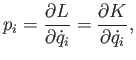(B.70)

where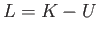is the Lagrangian of the system, and we have made use of the fact thatis independent of the. Consider the function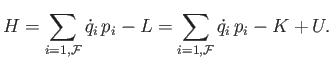(B.71)

If all of the conditions discussed previously are satisfied then Equations (B.69) and (B.70) yield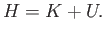(B.72)

In other words, the function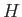is equal to the total energy of the system.

Consider the variation of the function. We have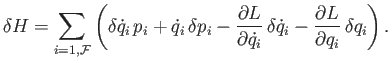(B.73)

The first and third terms in the bracket cancel, because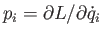. Furthermore, because Lagrange's equation can be written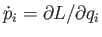(see Section B.4), we obtain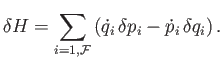(B.74)

Suppose, now, that we can express the total energy of the system,, solely as a function of theand the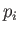, with no explicit dependence on the. In other words, suppose that we can write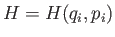. When the energy is written in this fashion it is generally termed the Hamiltonian of the system. The variation of the Hamiltonian function takes the form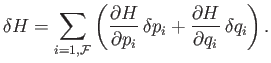(B.75)

A comparison of the previous two equations yields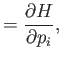(B.76)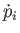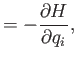(B.77)

for. These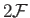first-order differential equations are known as Hamilton's equations. Hamilton's equations are often a useful alternative to Lagrange's equations, which take the form ofsecond-order differential equations.Next: Wave Mechanics Up: Classical Mechanics Previous: Hamilton's Principle
Richard Fitzpatrick 2016-01-25